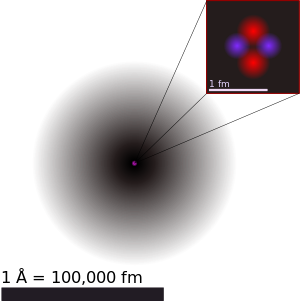# Can you estimate?

Chemistry Level 2The above is the diagram of a helium atom, showing the electron probability density as shades of gray. The atomic radius of a chemical element is a measure of the size of its atoms, usually the mean or typical distance from the nucleus to the boundary of the surrounding cloud of electrons. By the way, Lithium ($_3$Li), Beryllium ($_4$Be), Boron ($_5$B) and Carbon ($_6$C) have the same number of electron shells but different atomic radii. Which one has the largest atomic radius?

×